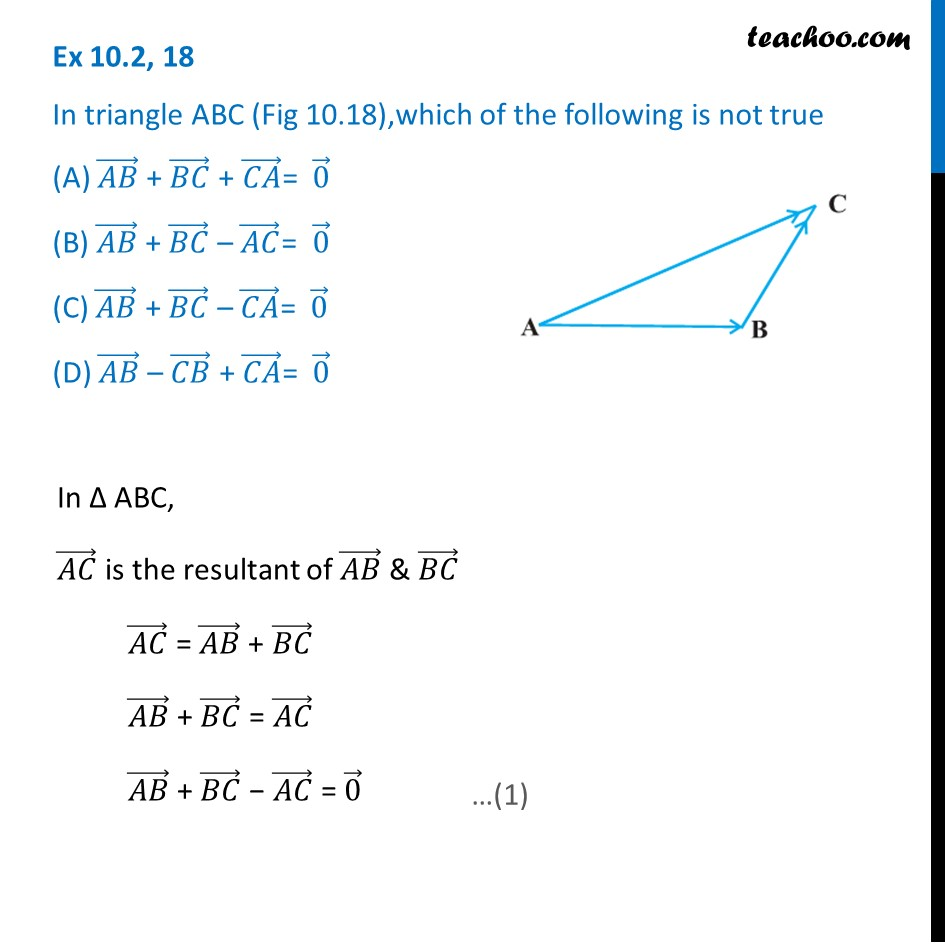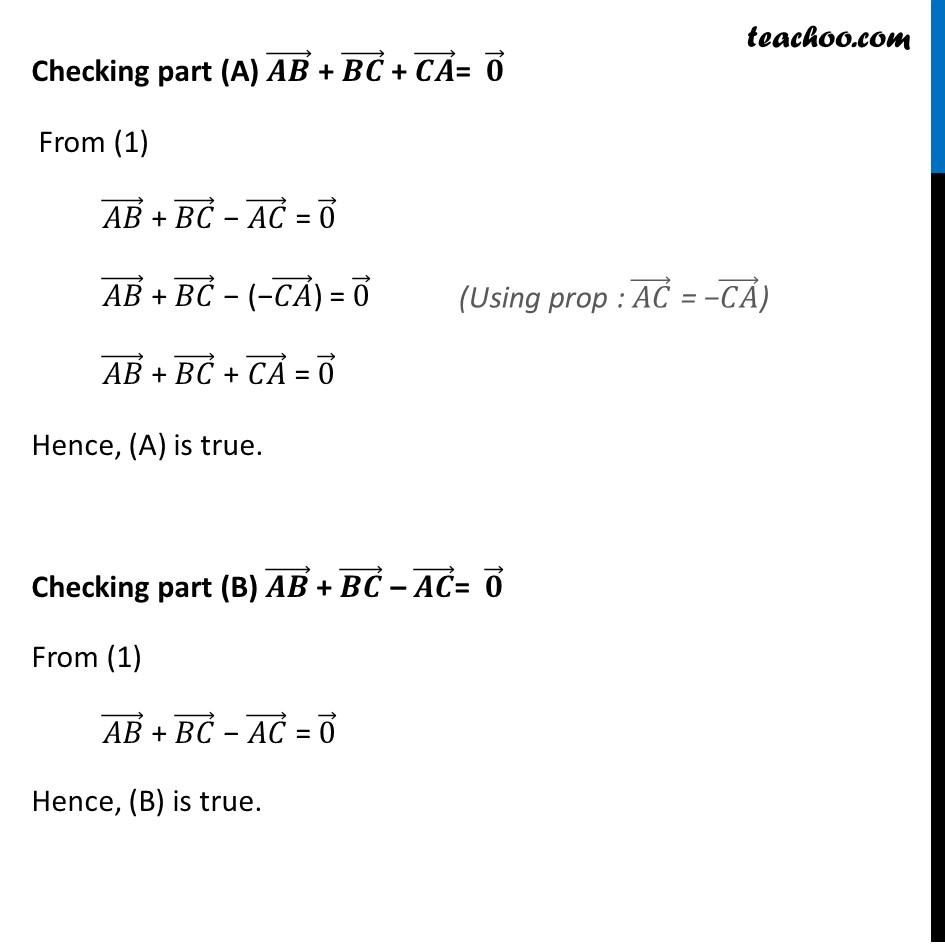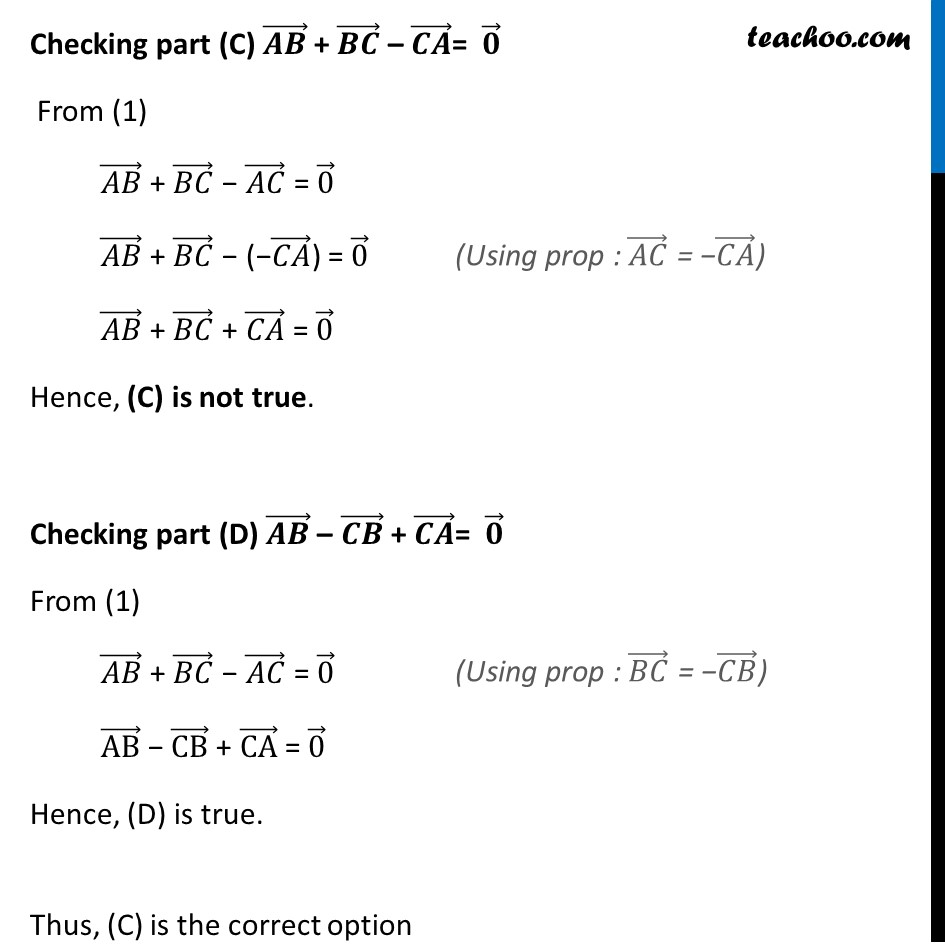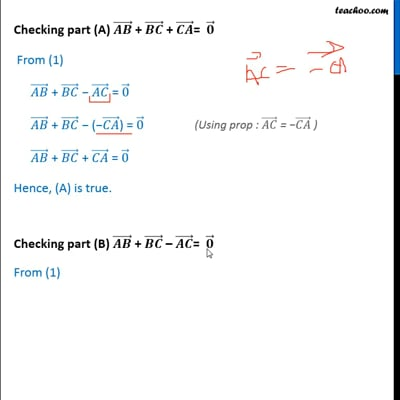Chapter 10 Class 12 Vector Algebra
Concept wiseThis video is only available for Teachoo black users

Introducing your new favourite teacher - Teachoo Black, at only ₹83 per month

### Transcript

Ex 10.2, 18 In triangle ABC (Fig 10.18),which of the following is not true (A) (𝐴𝐵) ⃗ + (𝐵𝐶) ⃗ + (𝐶𝐴) ⃗= 0 ⃗ (B) (𝐴𝐵) ⃗ + (𝐵𝐶) ⃗ – (𝐴𝐶) ⃗= 0 ⃗ (C) (𝐴𝐵) ⃗ + (𝐵𝐶) ⃗ – (𝐶𝐴) ⃗= 0 ⃗ (D) (𝐴𝐵) ⃗ – (𝐶𝐵) ⃗ + (𝐶𝐴) ⃗= 0 ⃗ In Δ ABC, (𝐴𝐶) ⃗ is the resultant of (𝐴𝐵) ⃗ & (𝐵𝐶) ⃗ (𝐴𝐶) ⃗ = (𝐴𝐵) ⃗ + (𝐵𝐶) ⃗ (𝐴𝐵) ⃗ + (𝐵𝐶) ⃗ = (𝐴𝐶) ⃗ (𝐴𝐵) ⃗ + (𝐵𝐶) ⃗ − (𝐴𝐶) ⃗ = 0 ⃗ Checking part (A) (𝑨𝑩) ⃗ + (𝑩𝑪) ⃗ + (𝑪𝑨) ⃗= 𝟎 ⃗ From (1) (𝐴𝐵) ⃗ + (𝐵𝐶) ⃗ − (𝐴𝐶) ⃗ = 0 ⃗ (𝐴𝐵) ⃗ + (𝐵𝐶) ⃗ − (−(𝐶𝐴) ⃗) = 0 ⃗ (𝐴𝐵) ⃗ + (𝐵𝐶) ⃗ + (𝐶𝐴) ⃗ = 0 ⃗ Hence, (A) is true. Checking part (B) (𝑨𝑩) ⃗ + (𝑩𝑪) ⃗ – (𝑨𝑪) ⃗= 𝟎 ⃗ From (1) (𝐴𝐵) ⃗ + (𝐵𝐶) ⃗ − (𝐴𝐶) ⃗ = 0 ⃗ Hence, (B) is true. Checking part (C) (𝑨𝑩) ⃗ + (𝑩𝑪) ⃗ – (𝑪𝑨) ⃗= 𝟎 ⃗ From (1) (𝐴𝐵) ⃗ + (𝐵𝐶) ⃗ − (𝐴𝐶) ⃗ = 0 ⃗ (𝐴𝐵) ⃗ + (𝐵𝐶) ⃗ − (−(𝐶𝐴) ⃗) = 0 ⃗ (𝐴𝐵) ⃗ + (𝐵𝐶) ⃗ + (𝐶𝐴) ⃗ = 0 ⃗ Hence, (C) is not true. Checking part (D) (𝑨𝑩) ⃗ – (𝑪𝑩) ⃗ + (𝑪𝑨) ⃗= 𝟎 ⃗ From (1) (𝐴𝐵) ⃗ + (𝐵𝐶) ⃗ − (𝐴𝐶) ⃗ = 0 ⃗ (AB) ⃗ − (CB) ⃗ + (CA) ⃗ = 0 ⃗ Hence, (D) is true. Thus, (C) is the correct option